Duncan Lardeau Juvenile Rainbow Trout Abundance 2020

The suggested citation for this analytic report is:

Thorley, J.L. and Amies-Galonski, E. (2020) Duncan Lardeau Juvenile Rainbow Trout Abundance 2020. A Poisson Consulting Analysis Appendix. URL: https://www.poissonconsulting.ca/f/723287510.

Background

Rainbow Trout rear in the Lardeau and Lower Duncan rivers. Since 2006 (with the exception of 2015) annual spring snorkel surveys have been conducted to estimate the abundance and distribution of age-1 Rainbow Trout. From 2006 to 2010 the surveys were conducted at fixed index sites. Since 2011 fish observations have been mapped to the river based on their spatial coordinates as recorded by GPS.

The primary aims of the current analyses were to:

• Estimate the spring abundance of age-1 fish by year.
• Estimate the egg deposition.
• Estimate the stock-recruitment relationship between the egg deposition and the abundance of age-1 recruits the following spring.
• Estimate the survival from age-1 to age-2.

Methods

Data Preparation

The data were provided by the Ministry of Forests, Lands and Natural Resource Operations (MFLNRO). The historical and current snorkel count data were manipulated using R version 4.0.3 and organised in an SQLite database.

Data Analysis

Hierarchical Bayesian models were fitted to the data using R version 4.0.3 , Stan 2.16.0 and JAGS 4.2.0 and the mbr family of packages.

Unless indicated otherwise, the models used prior distributions that were vague in the sense that they did not affect the posterior distributions . The posterior distributions were estimated from 2,000 Markov Chain Monte Carlo (MCMC) samples thinned from the second halves of three chains . Model convergence was confirmed by ensuring that $$\hat{R} < 1.1$$ for each of the monitored parameters in the model . Where relevant, model adequacy was confirmed by examination of residual plots.

The posterior distributions of the fixed parameters are summarised in terms of the point estimate, standard deviation (sd), the z-score, lower and upper 95% confidence/credible limits (CLs) and the p-value . The estimate is the median (50th percentile) of the MCMC samples, the z-score is $$\mathrm{sd}/\mathrm{mean}$$ and the 95% CLs are the 2.5th and 97.5th percentiles. A p-value of 0.05 indicates that the lower or upper 95% CL is 0.

Variable selection was achieved by dropping fixed variables with two-sided p-values $$\geq$$ 0.05 and random variables with percent relative errors $$\geq$$ 80%.

The results are displayed graphically by plotting the modeled relationships between particular variables and the response with 80% or 95% credible intervals (CIs) with the remaining variables held constant. In general, continuous and discrete fixed variables are held constant at their mean and first level values respectively while random variables are held constant at their typical values (expected values of the underlying hyperdistributions) . Where informative the influence of particular variables is expressed in terms of the effect size (i.e., percent change in the response variable) with 95% CIs .

Model Descriptions

Length Correction

The annual bias (inaccuracy) and error (imprecision) in observer’s fish length estimates when spotlighting (standing) and snorkeling were quantified from the divergence of their length distribution from the length distribution for all observers (including measured fish) in that year. More specifically, the length correction that minimised the Jensen-Shannon divergence (Lin 1991) between the two distributions provided a measure of the inaccuracy while the minimum divergence (the Jensen-Shannon divergence was calculated with log to base 2 which means it lies between 0 and 1) provided a measure of the imprecision.

After correcting the fish lengths, age-1 individuals were assumed to be those with a fork length $$\leq$$ 100 mm.

Abundance

The abundance was estimated from the count data using an overdispersed Poisson model . The annual abundance estimates represent the total number of fish in the study area.

Key assumptions of the abundance model include:

• The lineal fish density varies with year, useable width and river kilometer as a polynomial, and randomly with site.
• The observer efficiency at marking sites varies by study design (GPS versus Index).
• The observer efficiency also varies by visit type (marking versus count) within study design and randomly by snorkeller.
• The expected count at a site is the expected lineal density multiplied by the site length, the observer efficiency and the proportion of the site surveyed.
• The residual variation in the actual count is gamma-Poisson distributed.

Condition

The condition of fish with a fork length $$\geq$$ 500 mm was estimated via an analysis of mass-length relations .

More specifically the model was based on the allometric relationship

$W = \alpha_c L^{\beta_c}$

where $$W$$ is the weight (mass), $$\alpha_c$$ is the coefficent, $$\beta_c$$ is the exponent and $$L$$ is the length.

To improve chain mixing the relation was log-transformed, i.e.,

$\log(W) = \log(\alpha_c) + \beta_c \log(L).$

Key assumptions of the condition model include:

• $$\alpha_c$$ can vary randomly by year.
• The residual variation in weight is log-normally distributed.

Fecundity

The fecundity of females with a fork length $$\geq$$ 500 mm was estimated via an analysis of fecundity-mass relations.

More specifically the model was based on the allometric relationship

$F = \alpha_f W^{\beta_f}$

where $$F$$ is the fecundity, $$\alpha_f$$ is the coefficent, $$\beta_f$$ is the exponent and $$W$$ is the weight.

To improve chain mixing the relation was log-transformed.

Key assumptions of the fecundity model include:

• The residual variation in fecundity is log-normally distributed.

Spawner Size

The average length of the spawners in each year (for years for which it was unavailable) was estimated from the mean weight of Rainbow Trout in the Kootenay Lake Rainbow Trout Mailout Survey (KLRT) using a linear regression. This approach was suggested by Rob Bison.

Egg Deposition

The egg deposition in each year was estimated by

1. converting the average length of spawners to the average weight using the condition relationship for a typical year
2. adjusting the average weight by the annual condition effect (interpolating where unavailable)
3. converting the average weight to the average fecundity using the fecundity relationship
4. multiplying the average fecundity by the AUC based estimate of the number of females (assuming a sex ratio of 1:1)

Stock-Recruitment

The relationship between the number of eggs ($$E$$) and the abundance of age-1 individuals the following spring ($$R$$) was estimated using a Beverton-Holt stock-recruitment model :

$R = \frac{\alpha_s \cdot E}{1 + \beta_s \cdot E} \quad,$

where $$\alpha_s$$ is the maximum number of recruits per egg (egg survival), and $$\beta_s$$ is the density dependence.

Key assumptions of the stock-recruitment model include:

• The residual variation in the number of recruits is log-normally distributed with the standard deviation scaling with the uncertainty in the number of recruits.

The age-1 carrying capacity ($$K$$) is given by:

$K = \frac{\alpha_s}{\beta_s} \quad.$ and the $$E_{K/2}$$ Limit Reference Point ($$E_{0.5 R_{max}}$$), which corresponds to the stock (number of eggs) that produce 50% of the maximum recruitment ($$K$$), by $E_{K/2} = \frac{1}{\beta_s}$

The LRP was also converted into a number of spawners in a typical year (assuming 6,000 eggs per spawner and a sex ratio of 1:1).

Age-1 to Age-2 Survival

The relationship between the number of age-1 individuals and the number of age-2 individuals the following year was estimated using a linear regression through the origin where the slope was constrained to lie between 0 and 1 by a logistic transformation.

Key assumptions of the survival rate model include:

• The residual variation in the number of age-2 individuals is log-normally distributed.

Results

Model Templates

Abundance

data {

int<lower=0> nMarked;
int<lower=0> Marked[nMarked];
int<lower=0> Resighted[nMarked];
int<lower=0> IndexMarked[nMarked];

int<lower=0> nObs;
int Marking[nObs];
int Index[nObs];
int<lower=0> nSwimmer;
int<lower=0> Swimmer[nObs];
int<lower=0> nYear;
int<lower=0> Year[nObs];
real Rkm[nObs];
int<lower=0> nSite;
int<lower=0> Site[nObs];

real SiteLength[nObs];
real SurveyProportion[nObs];

int Count[nObs];
}
parameters {
real bEfficiency;
real bEfficiencyIndex;

real bDensity;
real<lower=0> sDensityYear;
vector[nYear] bDensityYear;

vector bDensityRkm;
real<lower=0> sDensitySite;
vector[nSite] bDensitySite;

real bEfficiencyMarking;
real bEfficiencyMarkingIndex;

real<lower=0,upper=5> sEfficiencySwimmer;
vector[nSwimmer] bEfficiencySwimmer;

real<lower=0> sDispersion;
}
model {

vector[nObs] eDensity;
vector[nObs] eEfficiency;
vector[nObs] eAbundance;
vector[nObs] eCount;

sDispersion ~ gamma(0.01, 0.01);

bDensity ~ normal(0, 2);
bDensityRkm ~ normal(0, 2);
sDensitySite ~ uniform(0, 5);
bDensitySite ~ normal(0, sDensitySite);
sDensityYear ~ uniform(0, 5);
bDensityYear ~ normal(0, sDensityYear);

bEfficiency ~ normal(0, 5);
bEfficiencyIndex ~ normal(0, 5);
bEfficiencyMarking ~ normal(0, 5);
bEfficiencyMarkingIndex ~ normal(0, 5);
sEfficiencySwimmer ~ uniform(0, 5);
bEfficiencySwimmer ~ normal(0, sEfficiencySwimmer);

for (i in 1:nMarked) {
target += binomial_lpmf(Resighted[i] | Marked[i],
inv_logit(
bEfficiency +
bEfficiencyIndex * IndexMarked[i] +
bEfficiencyMarking +
bEfficiencyMarkingIndex * IndexMarked[i]
));
}

for (i in 1:nObs) {
eDensity[i] = exp(bDensity +
bDensityRkm * Rkm[i] +
bDensityRkm * pow(Rkm[i], 2.0) +
bDensityRkm * pow(Rkm[i], 3.0) +
bDensityRkm * pow(Rkm[i], 4.0) +
bDensitySite[Site[i]] +
bDensityYear[Year[i]]);

eEfficiency[i] = inv_logit(
bEfficiency +
bEfficiencyIndex * Index[i] +
bEfficiencyMarking * Marking[i] +
bEfficiencyMarkingIndex * Index[i] * Marking[i] +
bEfficiencySwimmer[Swimmer[i]]);

eAbundance[i] = eDensity[i] * SiteLength[i];

eCount[i] = eAbundance[i] * eEfficiency[i] * SurveyProportion[i];
}

target += neg_binomial_2_lpmf(Count | eCount, sDispersion);
}

Block 1. Abundance model description.

Condition

data {
int nYear;
int nObs;

vector[nObs] Length;
vector[nObs] Weight;
int Year[nObs];

parameters {
real bWeight;
real bWeightLength;
real sWeightYear;

vector[nYear] bWeightYear;
real sWeight;

model {

vector[nObs] eWeight;

bWeight ~ normal(-10, 5);
bWeightLength ~ normal(3, 2);

sWeightYear ~ normal(-2, 5);

for (i in 1:nYear) {
bWeightYear[i] ~ normal(0, exp(sWeightYear));
}

sWeight ~ normal(-2, 5);
for(i in 1:nObs) {
eWeight[i] = bWeight + bWeightLength * log(Length[i]) + bWeightYear[Year[i]];
Weight[i] ~ lognormal(eWeight[i], exp(sWeight));
}

Block 2.

Fecundity

data {
int nObs;

vector[nObs] Weight;
vector[nObs] Fecundity;

parameters {
real bFecundity;
real bFecundityWeight;
real sFecundity;

model {

vector[nObs] eFecundity;

bFecundity ~ uniform(0, 5);
bFecundityWeight ~ uniform(0, 2);

sFecundity ~ uniform(0, 1);
for(i in 1:nObs) {
eFecundity[i] = log(bFecundity) + bFecundityWeight * log(Weight[i]);
Fecundity[i] ~ lognormal(eFecundity[i], sFecundity);
}

Block 3.

Stock-Recruitment

model {
a ~ dunif(0, 1)
b ~ dunif(0, 0.1)
sScaling ~ dunif(0, 5)

eRecruits <- a * Stock / (1 + Stock * b)

for(i in 1:nObs) {
esRecruits[i] <- SDLogRecruits[i] * sScaling
Recruits[i] ~ dlnorm(log(eRecruits[i]), esRecruits[i]^-2)
}

Block 4. Stock-Recruitment model description.

Age-1 to Age-2 Survival

model {
bSurvival ~ dnorm(0, 2^-2)
sRecruits ~ dnorm(0, 2^-2) T(0,)

for(i in 1:nObs) {
logit(eSurvival[i]) <- bSurvival
eRecruits[i] <- Stock[i] * eSurvival[i]
Recruits[i] ~ dlnorm(log(eRecruits[i]), sRecruits^-2)
}

Block 5. In-river survival model description.

Tables

Abundance

Table 1. Parameter descriptions.

Parameter Description
bDensity Intercept for log(eDensity)
bDensityRkm[i] ith-order polynomial coefficients of effect of river kilometer on bDensity
bDensitySite[i] Effect of ith Site on bDensity
bDensityYear[i] Effect of ith Year on bDensity
bEfficiency Intercept of logit(eEfficiency)
bEfficiencyIndex Effect of Index on bEfficiency
bEfficiencyMarking Effect of Marking on bEfficiency
bEfficiencyMarkingIndex Effect of Marking and Index on bEfficiency
bEfficiencySwimmer[i] Effect of ith Swimmer on bEfficiency
eAbundance[i] Expected abundance of fish at site of ith visit
eCount[i] Expected total number of fish at site of ith visit
eDensity[i] Expected lineal density of fish at site of ith visit
eEfficiency[i] Expected observer efficiency on ith visit
Index Whether the ith visit was to an index site
Marking[i] Whether the ith visit was to a site with marked fish
Rkm[i] River kilometer of ith visit
sDensitySite SD of bDensitySite
sDispersion Overdispersion of Count[i]
sEfficiencySwimmer SD of bEfficiencySwimmer
Site[i] Site of ith visit
SiteLength[i] Length of site of ith visit
SurveyProportion[i] Proportion of site surveyed on ith visit
Swimmer[i] Snorkeler on ith site visit
Year[i] Year of ith site visit
Rainbow Trout
Age1

Table 2. Model coefficients.

term estimate sd zscore lower upper pvalue
bDensity -1.1025638 0.2580144 -4.2599319 -1.6027172 -0.6136417 0.0019987
bDensityRkm -0.2361983 0.0758283 -3.1184009 -0.3873395 -0.0806590 0.0019987
bDensityRkm 0.5633199 0.1085001 5.2044144 0.3485749 0.7706941 0.0006662
bDensityRkm -0.0802012 0.0354745 -2.2803441 -0.1513637 -0.0101689 0.0193205
bDensityRkm -0.2714619 0.0374247 -7.2865624 -0.3451534 -0.2005796 0.0006662
bEfficiency -1.7859982 0.1443442 -12.3573110 -2.0662570 -1.5030707 0.0006662
bEfficiencyIndex 0.2914821 0.3214882 0.8978293 -0.3129780 0.9413404 0.3630913
bEfficiencyMarking 0.5304248 0.1108045 4.7976614 0.3084469 0.7500068 0.0006662
bEfficiencyMarkingIndex 0.9202822 0.3272740 2.7680477 0.2469951 1.5173923 0.0099933
sDensitySite 0.6568752 0.0373079 17.6013912 0.5881179 0.7321685 0.0006662
sDensityYear 0.6530751 0.1647549 4.1456660 0.4330231 1.0561532 0.0006662
sDispersion 1.3177023 0.0683175 19.3231229 1.1870013 1.4562109 0.0006662
sEfficiencySwimmer 0.5299685 0.1577689 3.5069406 0.3041078 0.9240397 0.0006662

Table 3. Model summary.

n K nchains niters nthin ess rhat converged
3274 13 3 500 5 747 1.008 TRUE
Age2

Table 4. Model coefficients.

term estimate sd zscore lower upper pvalue
bDensity -2.0765922 0.2742946 -7.5395655 -2.5673257 -1.5274241 0.0006662
bDensityRkm -0.1888056 0.0940985 -2.0300594 -0.3717058 -0.0085009 0.0406396
bDensityRkm 0.0453724 0.1346785 0.3428431 -0.2120224 0.3123082 0.7521652
bDensityRkm 0.0410407 0.0441837 0.9106852 -0.0469177 0.1261699 0.3617588
bDensityRkm -0.1026092 0.0459050 -2.2572536 -0.1939733 -0.0158174 0.0246502
bEfficiency -1.9859859 0.2323886 -8.5416723 -2.4486317 -1.5216252 0.0006662
bEfficiencyIndex 0.4838928 0.3592518 1.3408354 -0.2059524 1.1835048 0.1725516
bEfficiencyMarking 0.7299017 0.1735978 4.2100673 0.3752527 1.0646317 0.0006662
bEfficiencyMarkingIndex 2.1789624 0.5643068 3.8936001 1.1281441 3.3214454 0.0006662
sDensitySite 0.7595852 0.0482679 15.7839776 0.6678163 0.8601676 0.0006662
sDensityYear 0.5659470 0.1548924 3.8428078 0.3801633 0.9738893 0.0006662
sDispersion 1.1505705 0.0934440 12.3738105 0.9862979 1.3533862 0.0006662
sEfficiencySwimmer 0.3918160 0.1215545 3.4160466 0.2395297 0.6940196 0.0006662

Table 5. Model summary.

n K nchains niters nthin ess rhat converged
3274 13 3 500 5 748 1.006 TRUE

Condition

Table 6. Parameter descriptions.

Parameter Description
bWeight Intercept of log(eWeight)
bWeightLength Intercept of effect of log(Length) on bWeight
bWeightYear[i] Effect of ith Year on bWeight
eWeight[i] Expected Weight of ith fish
Length[i] Fork length of ith fish
sWeight Log standard deviation of residual variation in log(Weight)
sWeightYear Log standard deviation of bWeightYear
Weight[i] Recorded weight of ith fish
Year[i] Year ith fish was captured

Table 7. Model coefficients.

term estimate sd zscore lower upper pvalue
bWeight -12.640509 0.2124435 -59.49947 -13.044759 -12.232333 0.0006662
bWeightLength 3.202909 0.0320422 99.96166 3.140421 3.265140 0.0006662
sWeight -1.906869 0.0210349 -90.64072 -1.946943 -1.864212 0.0006662
sWeightYear -2.117223 0.1635939 -12.90748 -2.418278 -1.787881 0.0006662

Table 8. Model summary.

n K nchains niters nthin ess rhat converged
1113 4 3 500 2 339 1.016 TRUE

Fecundity

Table 9. Parameter descriptions.

Parameter Description
bFecundity Intercept of eFecundity
bFecundityWeight Effect of log(Weight) on log(bFecundity)
eFecundity[i] Expected Fecundity of ith fish
Fecundity[i] Fecundity of ith fish (eggs)
sFecundity SD of residual variation in log(Fecundity)
Weight[i] Weight of ith fish (mm)

Table 10. Model coefficients.

term estimate sd zscore lower upper pvalue
bFecundity 3.8623885 0.9616924 3.831203 1.5125461 4.9580463 0.0006662
bFecundityWeight 0.8609092 0.0364533 23.905887 0.8320917 0.9680592 0.0006662
sFecundity 0.1266241 0.0214832 6.007216 0.0949847 0.1758600 0.0006662

Table 11. Model summary.

n K nchains niters nthin ess rhat converged
22 3 3 500 4 413 1.004 TRUE

Stock-Recruitment

Table 12. Parameter descriptions.

Parameter Description
a Recruits per Stock at low density
b Density-dependence
eRecruits[i] Expected number of recruits from ith spawn year
esRecruits[i] Expected SD of residual variation in Recruits
Recruits[i] Number of recruits from ith spawn year
SDLogRecruits[i] Standard deviation of uncertainty in log(Recruits[i])
sScaling Scaling term for SD of residual variation in log(eRecruits)
Stock[i] Number of egg in ith spawn year
Age1

Table 13. Model coefficients.

term estimate sd zscore lower upper pvalue
a 0.5631954 0.1994569 2.949574 0.2615310 0.9619482 0.0006662
b 0.0000053 0.0000025 2.241339 0.0000019 0.0000112 0.0006662
sScaling 2.2449732 0.5204364 4.478682 1.5541732 3.6233816 0.0006662

Table 14. Model summary.

n K nchains niters nthin ess rhat converged
14 3 3 500 100 1479 1.001 TRUE

Table 15. Estimated carry capacity (with 95% CRIs).

estimate lower upper
109000 76400 161000

Table 16. Estimated reference points (with 80% CRIs).

Metric estimate lower upper
eggs 190000.00000 107000.00000 374000.0000
spawners 63.33333 35.66667 124.6667

Age-1 to Age-2 Survival

Table 17. Parameter descriptions.

Parameter Description
bSurvival logit(eSurvival)
eSurvival[i] Expected annual survival for ith spawn year
Recruits[i] Number of age-2 juveniles from ith spawn year
sRecruits SD of residual variation in Recruits
Stock[i] Number of age-1 juveniles from ith spawn year
Age2

Table 18. Model coefficients.

term estimate sd zscore lower upper pvalue
bSurvival -0.8802652 0.1962366 -4.429277 -1.2346200 -0.4522072 0.0006662
sRecruits 0.4398566 0.1258648 3.723528 0.3017872 0.7828409 0.0006662

Table 19. Model summary.

n K nchains niters nthin ess rhat converged
12 2 3 500 1 435 1.01 TRUE

Figures

Abundance

Age1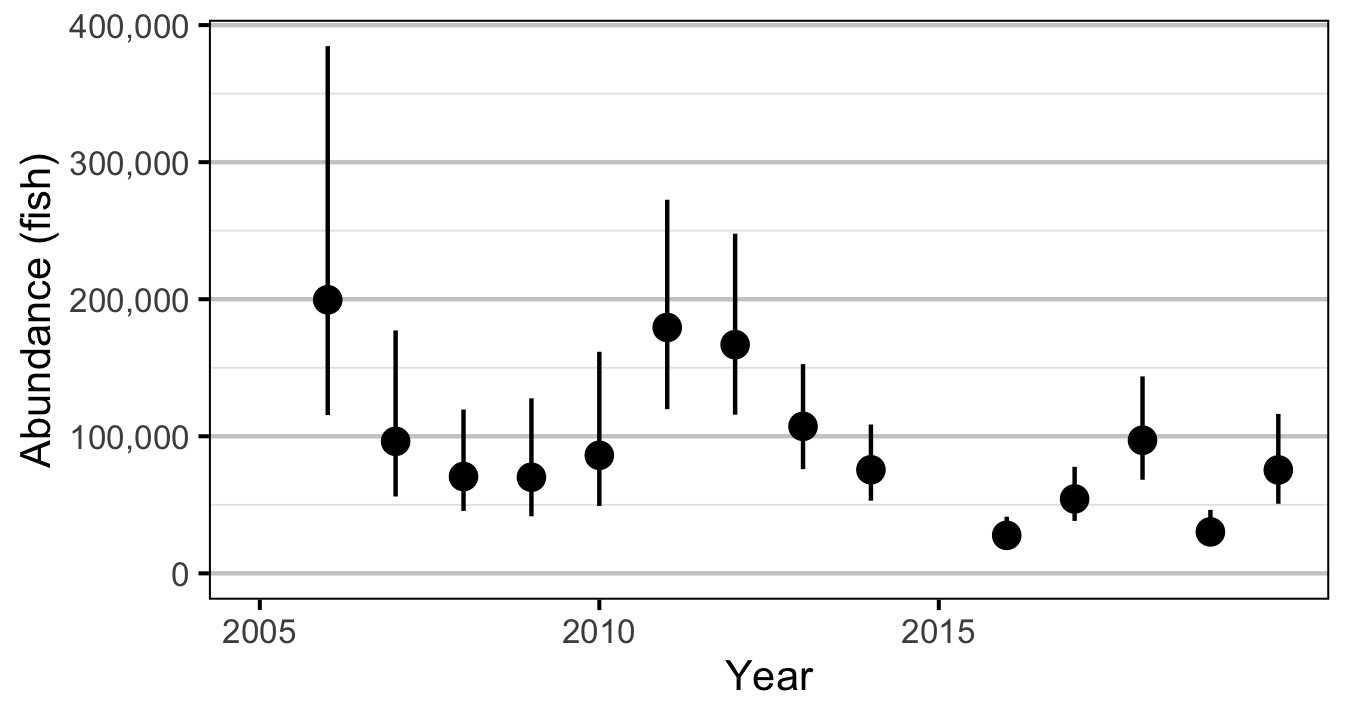Figure 3. Predicted abundance of age-1 Rainbow Trout in the Duncan and Lardeau Rivers by year (with 95% CRIs).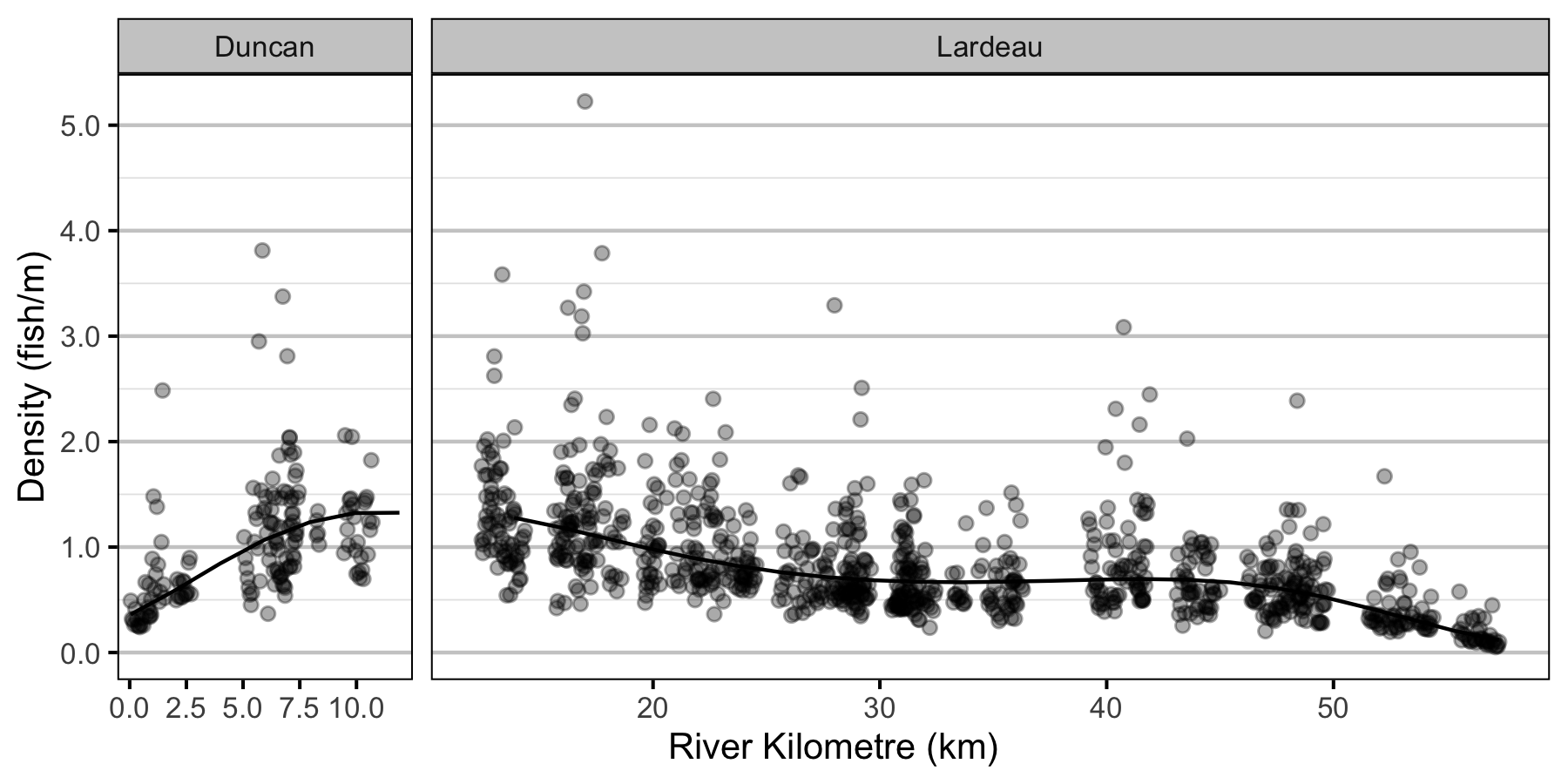Figure 4. Predicted lineal density of age-1 Rainbow Trout in 2010 by river kilometre (with 95% CRIs).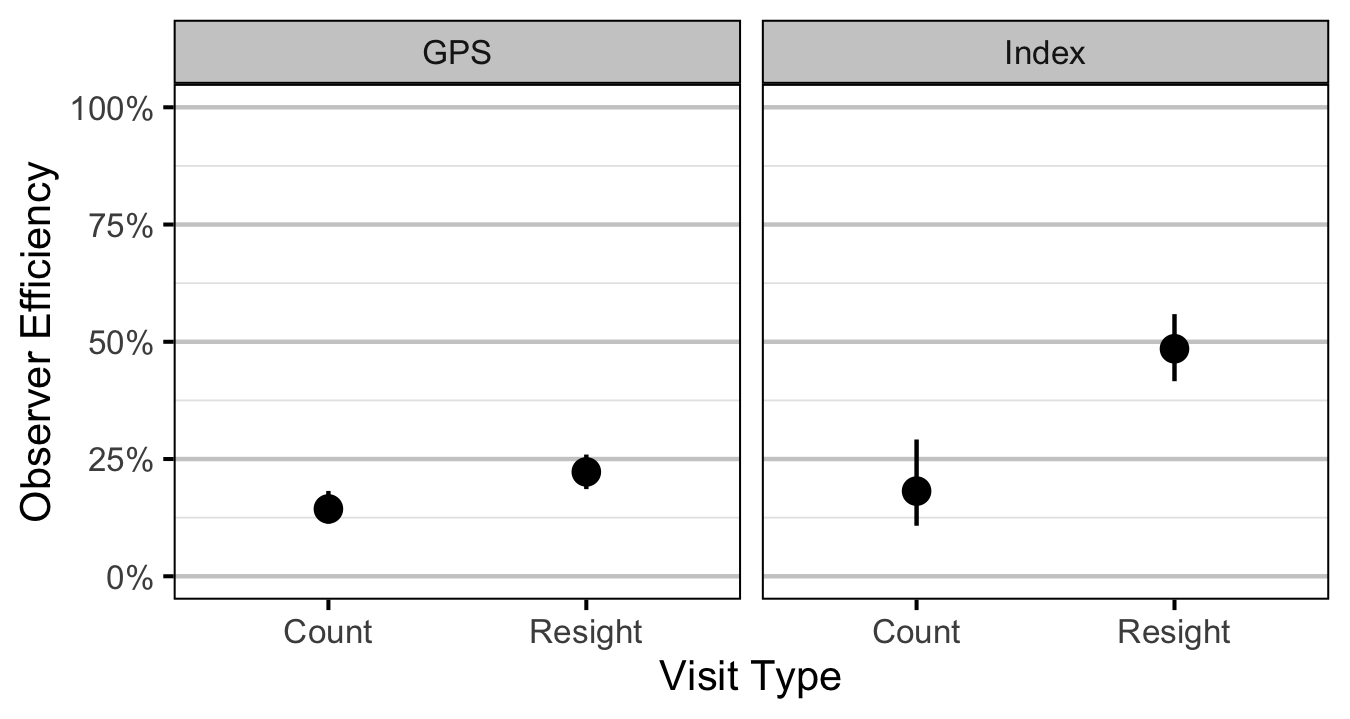Figure 5. Predicted observer efficiency for age-1 Rainbow Trout by visit type and study design (with 95% CRIs).
Age2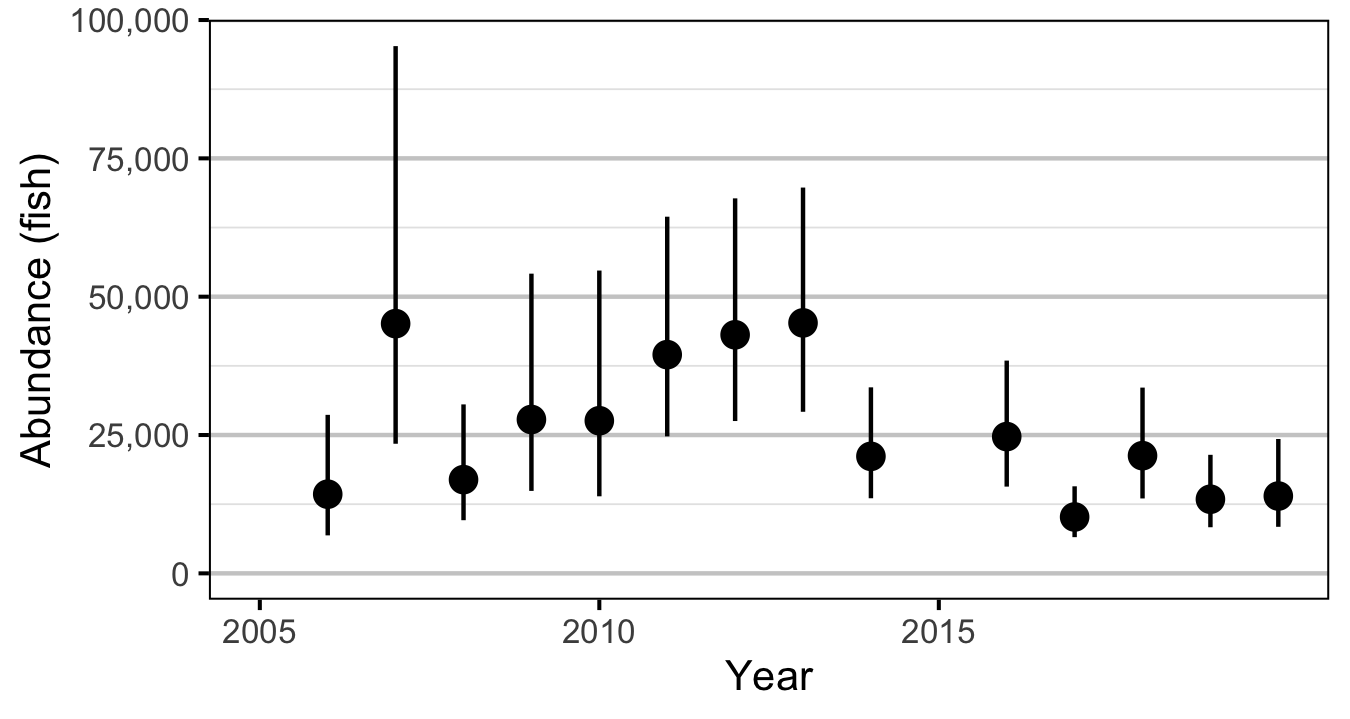Figure 6. Predicted abundance of age-2 Rainbow Trout in the Duncan and Lardeau Rivers by year (with 95% CRIs).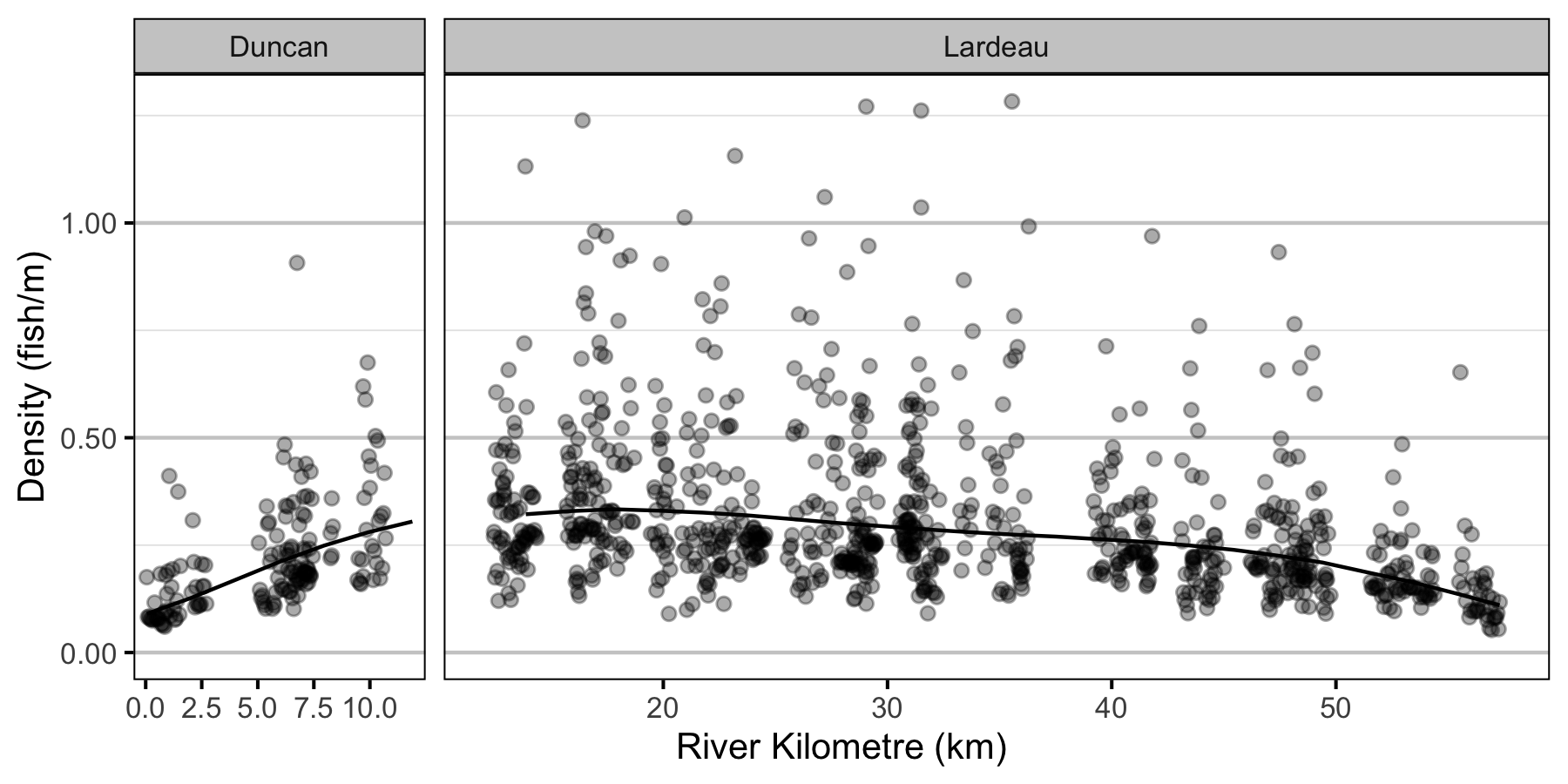Figure 7. Predicted lineal density of age-2 Rainbow Trout in 2010 by river kilometre (with 95% CRIs).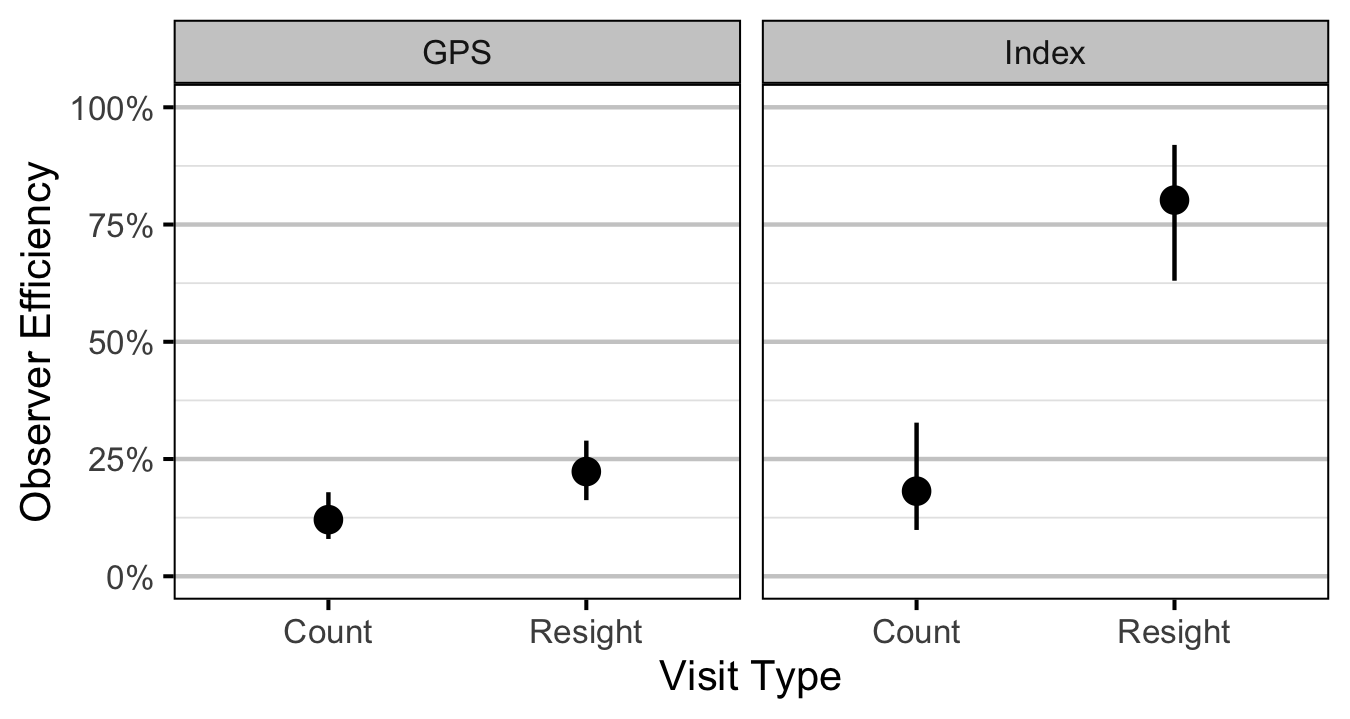Figure 8. Predicted observer efficiency for age-2 Rainbow Trout by visit type and study design (with 95% CRIs).

Condition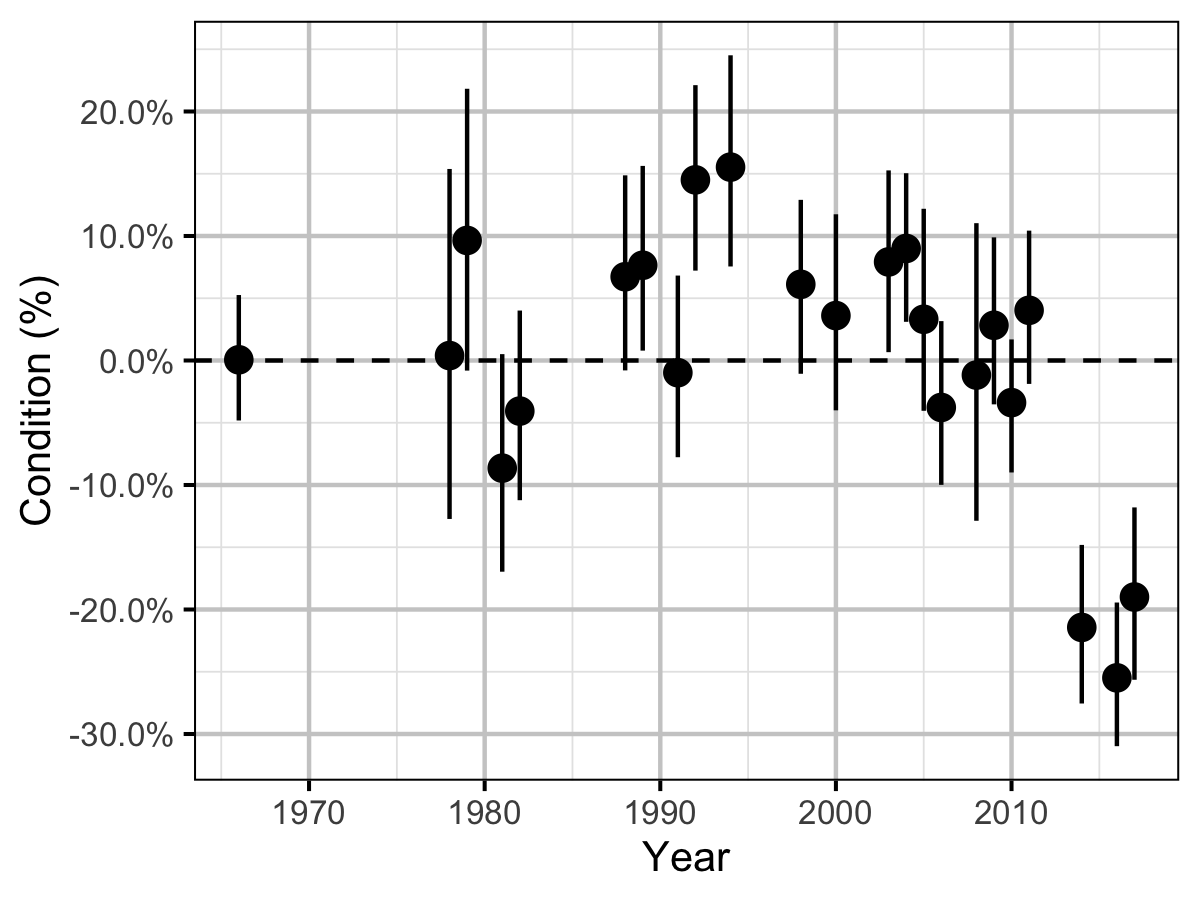Figure 9. The percent change in the body condition for an average length fish relative to a typical year by year (with 95% CRIs).

Spawner Size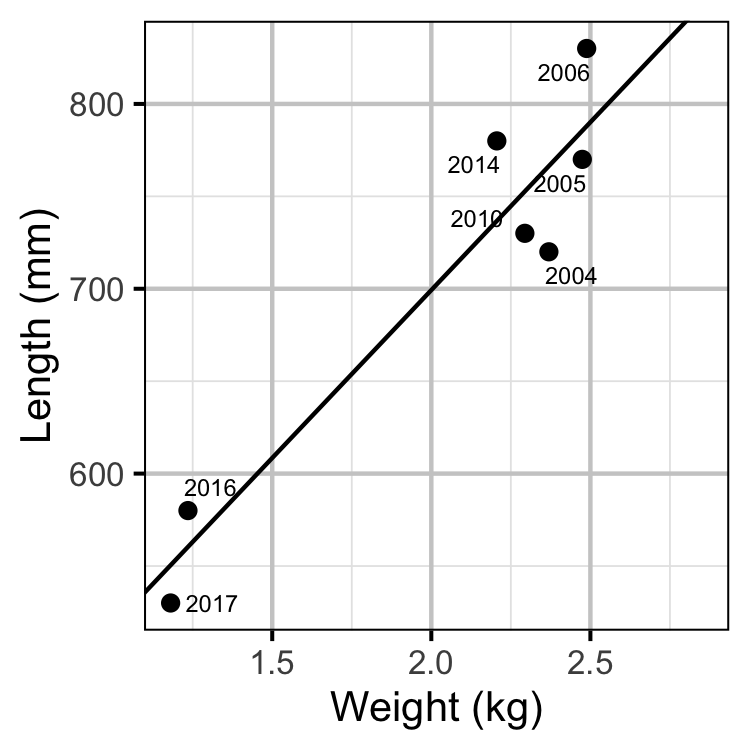Figure 11. The mean length of spawning Rainbow Trout by the mean weight of Rainbow Trout in the KLRT.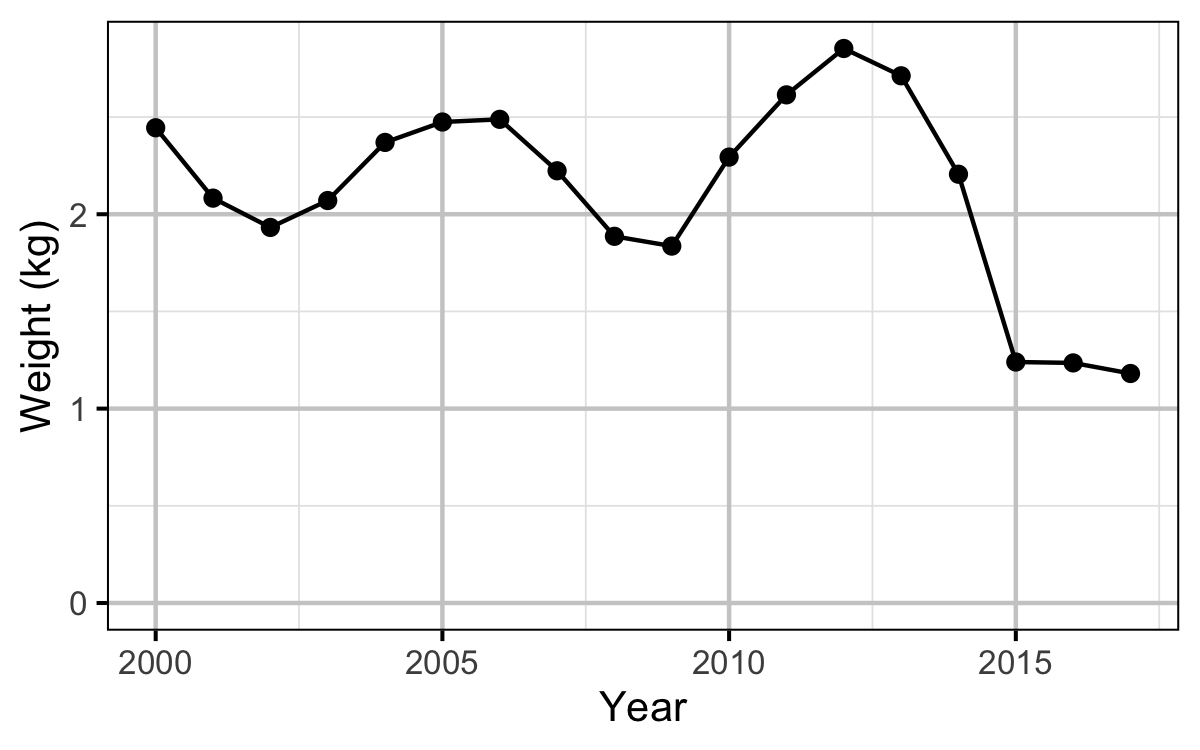Figure 12. The mean weight of Rainbow Trout in the KLRT by year.

Stock-Recruitment

Age1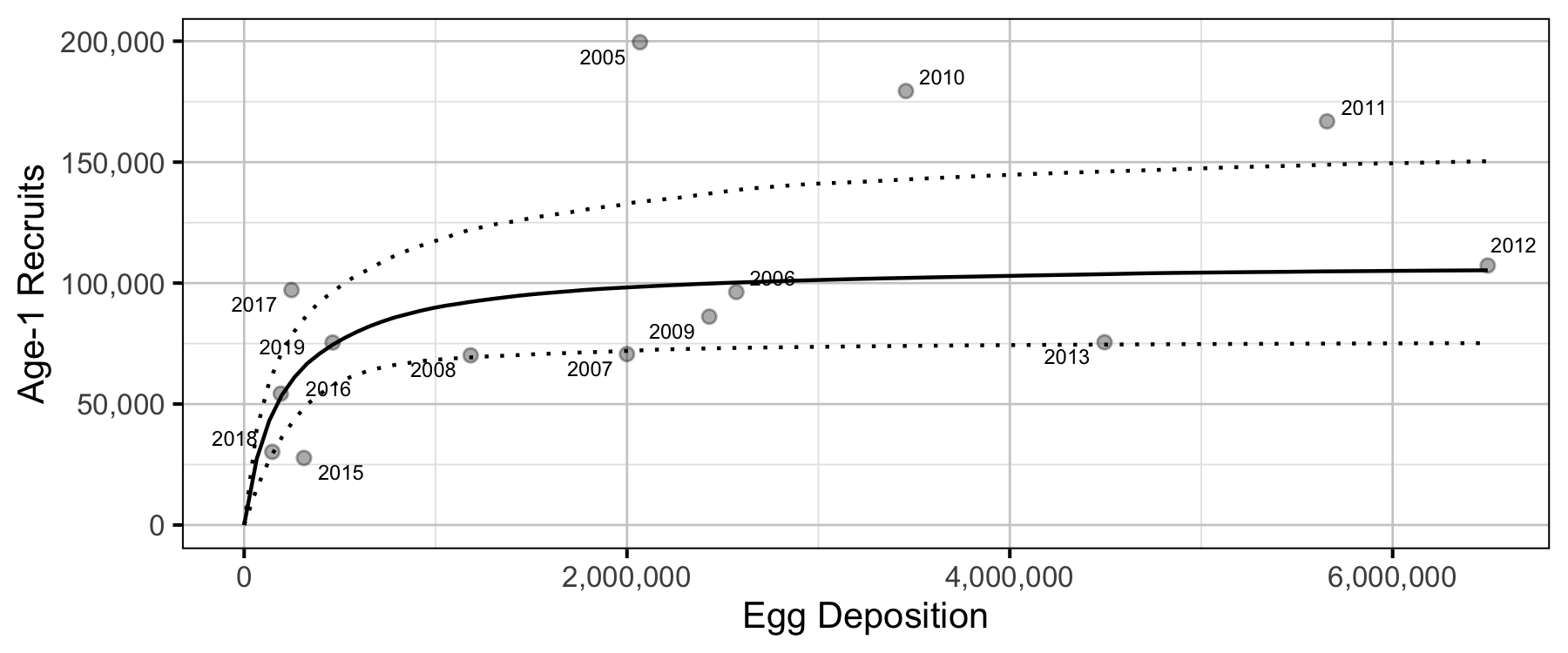Figure 16. Predicted stock-recruitment relationship between spawners and age-1 recruits (with 95% CRIs).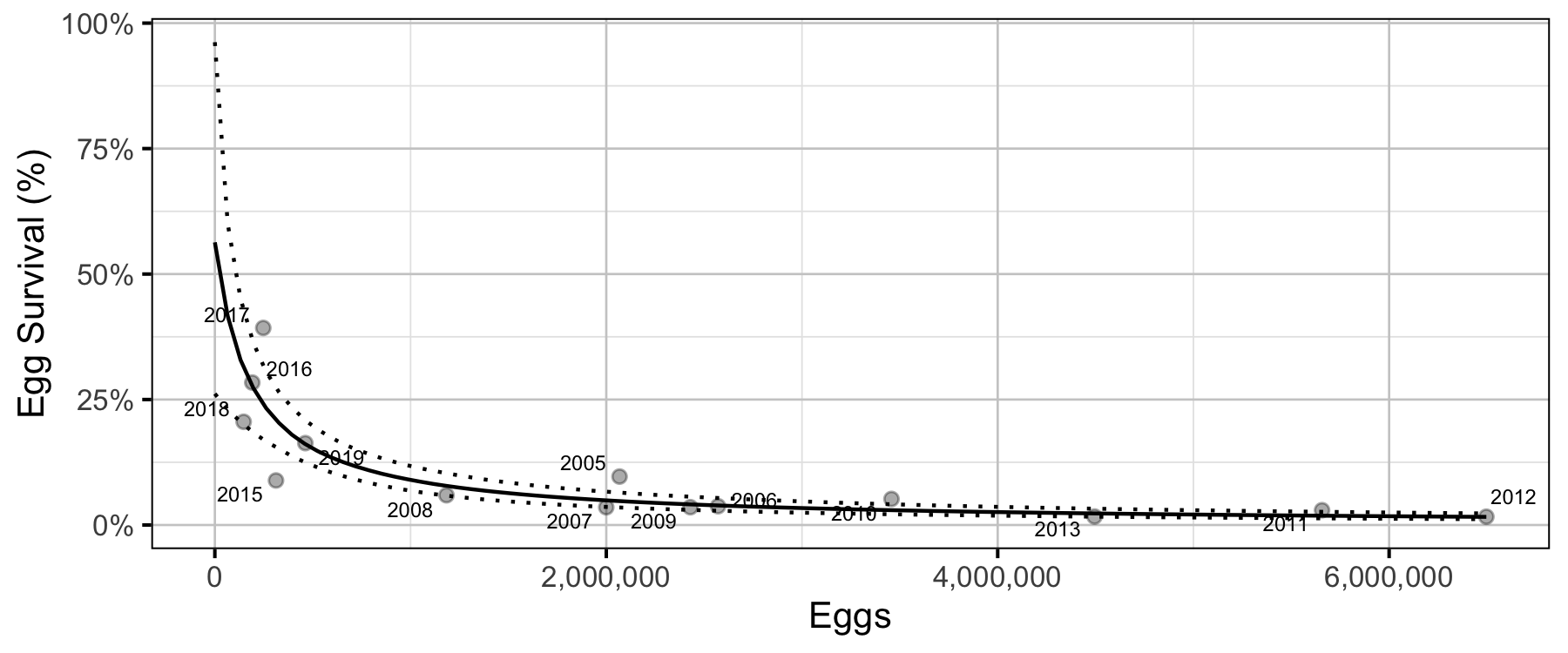Figure 17. Predicted egg survival to age-1 by egg deposition (with 95% CRIs).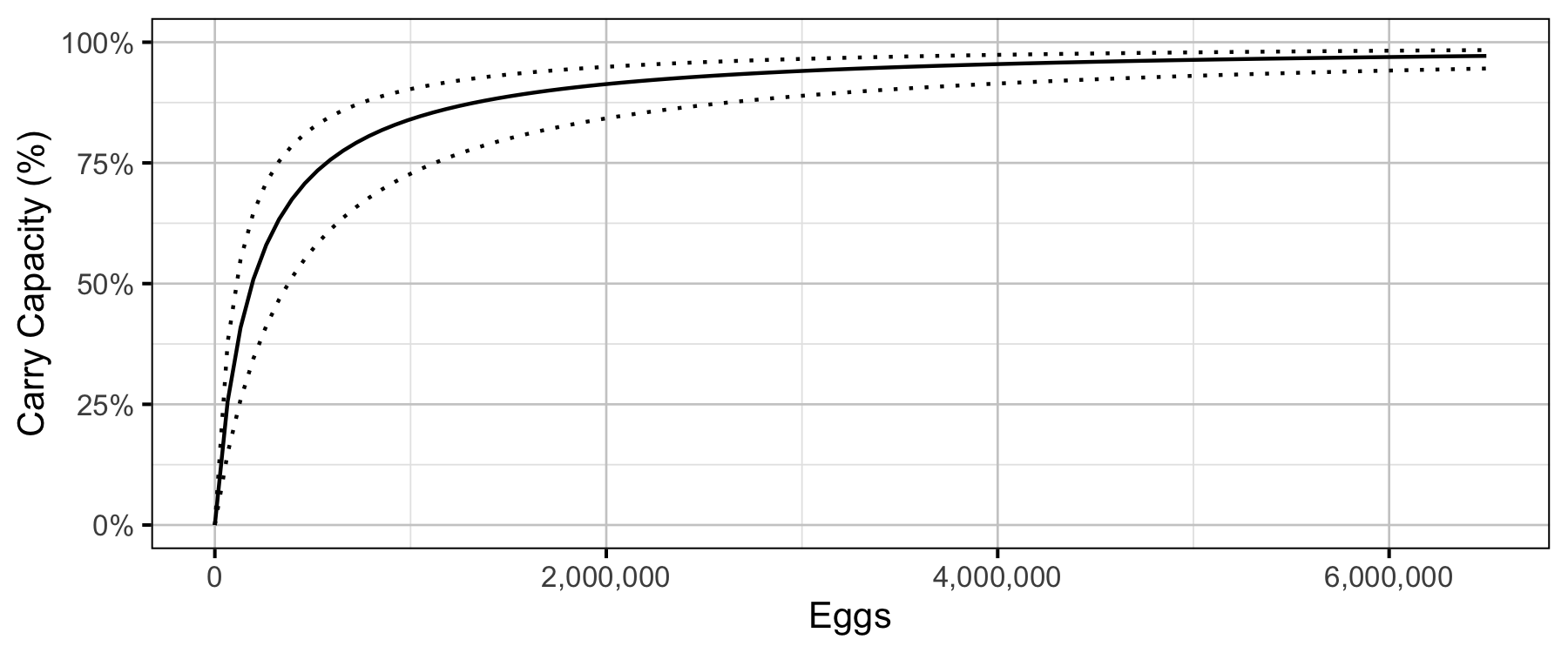Figure 18. Predicted percent of age-1 recruits carry capacity by egg deposition (with 80% CRIs).

Age-1 to Age-2 Survival

Age2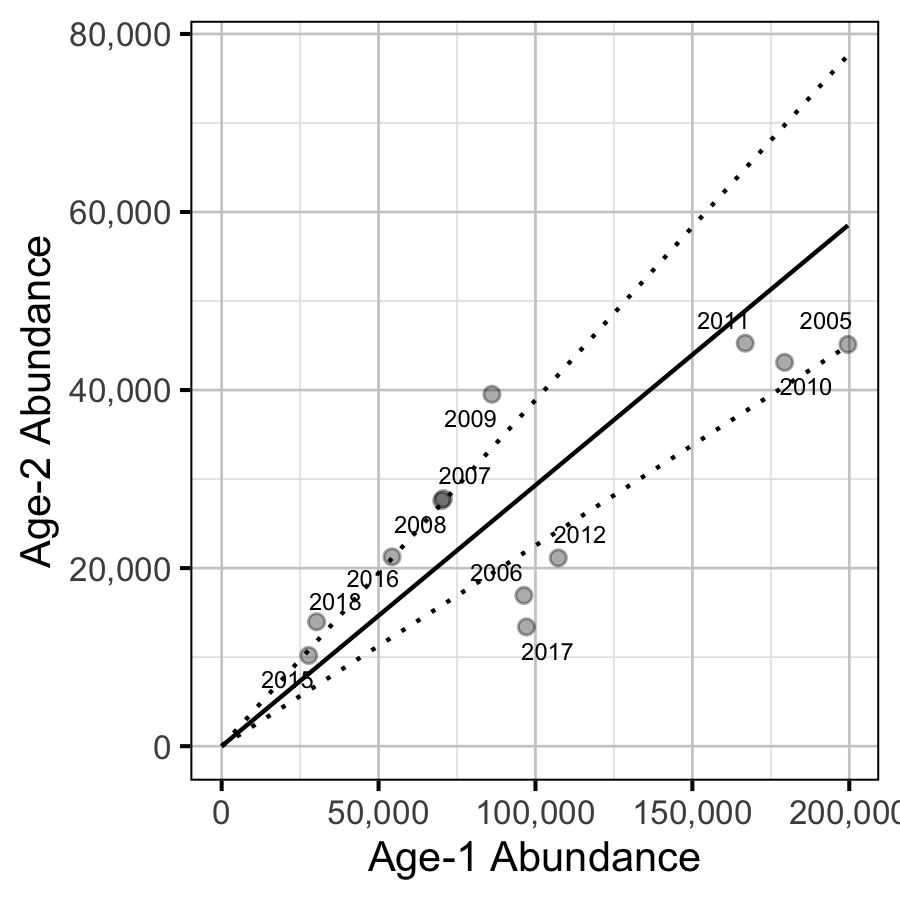Figure 19. Predicted relationship between age-1 and age-2 abundance (with 95% CRIs).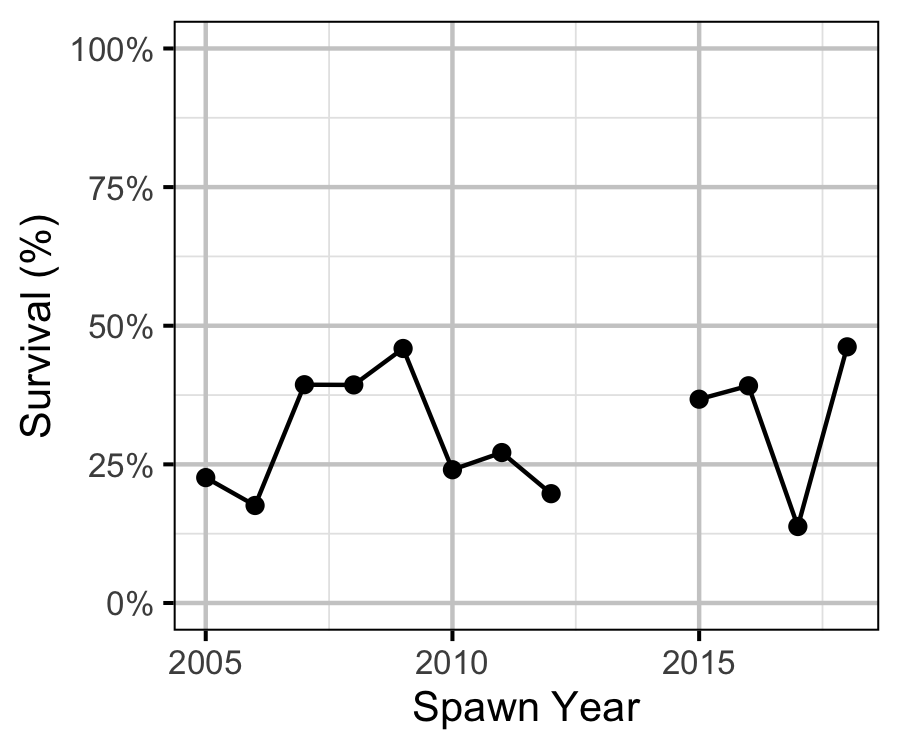Figure 20. Estimated age-1 and age-2 survival by spawn year.

Acknowledgements

The organisations and individuals whose contributions have made this analysis report possible include: